Students can download Maths Chapter 1 Numbers Ex 1.2 Questions and Answers, Notes, Samacheer Kalvi 6th Maths Guide Pdf helps you to revise the complete Tamilnadu State Board New Syllabus, helps students complete homework assignments and to score high marks in board exams.

## Tamilnadu Samacheer Kalvi 6th Maths Solutions Term 2 Chapter 1 Numbers Ex 1.2

Question 1.
Fill in the blanks
(i) The HCF of 45 and 75 is …….
(ii) The HCF of two successive even numbers is …….
(iii) If the LCM of 3 and 9 is 9, then their HCF is ………
(iv) The LCM of 26, 39 and 52 is ……..
(v) The least number that should be added to 57 so that the sum is exactly divisible by 2, 3, 4 and 5 is ………
Solution:
(i) 15
(ii) 2
(iii) 3
(iv) 156
(v) 3The Least common multiple (LCM) of 15 and 20 is 60.

Question 2.
Say True or False
(i) The numbers 57 and 69 are co-primes.
(ii) The HCF of 17 and 18 is 1.
(iii) The LCM of two successive numbers is the product of the numbers.
(iv) The LCM of two co-primes is the sum of the numbers.
(v) The HCF of two numbers is always a factor of their LCM.
Solution:
(i) False
(ii) True
(iii) True
(iv) False
(v) TrueSo, now the prime factorization of 84 with the upside-down division method is 2 × 2 × 3 × 7.

Question 3.
Find the HCF of each set of numbers using the prime factorisation method.
(i) 18, 24
(ii) 51, 85
(iii) 61, 76
(iv) 84, 120
(v) 27, 45, 81
(vi) 45, 55, 95
Solution:
(i) 18, 24HCF = 2 × 3 = 6

(ii) 51, 85HCF = 17(iii) 61, 76
61 = 1 × 61
For 61, 76, the common factor is 1
HCF = 1

(iv) 84, 120HCF = 2 × 2 × 3 = 12

(v) 27, 45, 81HCF = 3 × 3 = 9

(vi) 45, 55, 95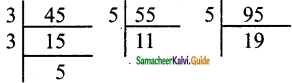Factors of 55 = 5 × 11
Factors of 45 = 3 × 3 × 5
Factors of 95 = 5 × 19
HCF = 5Question 4.
Find the LCM of each set of numbers using the prime factorisation method.
(i) 6, 9
(ii) 8, 12
(iii) 10, 15
(iv) 14, 42
(v) 30, 40, 60
(vi) 15, 25, 75
Solution:
(i) 6, 9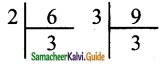Factors of 6 = 2 × 3
Factors of 9 = 3 × 3
LCM of 6 and 9 = 3 × 2 × 3 = 18

(ii) 8, 12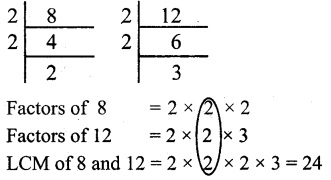(iii) 10, 15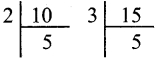Factors of 10 = 2 × 5
Factors of 15 = 3 × 5
LCM of 10 and 15 = 5 × 2 × 3 = 30(iv) 14, 42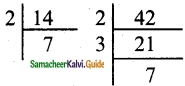Factors of 14 = 2 × 7
Factors of 42 = 2 × 3 × 7
LCM of 14 and 42 = 7 × 2 × 3 = 42

(v) 30, 40, 60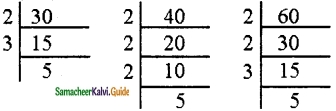Factors of 30 = 2 × 3 × 5
Factors of 40 = 2 × 2 × 2 × 5
Factors of 60 = 2 × 2 × 3 × 5
LCM of 30, 40, 60 = 2 × 5 × 3 × 2 × 2 = 120

(vi) 15, 25, 75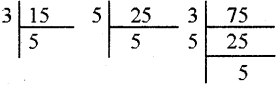Factors of 15 = 3 × 5
Factors of 25 =5 × 5
Factors of 75 = 3 × 5 × 5
LCM of 15, 25, 75 = 5 × 3 × 5 = 75Question 5.
Find the HCF and the LCM of the numbers 154, 198, 286
Solution:
HCF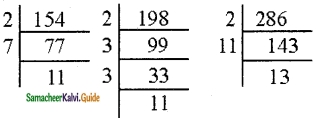Factors of 154 = 2 × 7 × 11
Factors of 198 = 2 × 3 × 3 × 11
Factors of 286 = 2 × 13 × 11
HCF of 154, 198, 286 = 11 × 2 = 22
LCM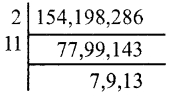LCM = 2 × 11 × 7 × 9 × 13
= 22 × 63 × 13
= 18018Question 6.
What is the greatest possible volume of a vessel that can be used to measure exactly the volume of milk in cans (in full capacity) of 80 litres, 100 litres and 120 litres?
Solution:
This is an HCF related problem. So, we need to find the HCF of 80, 100, 120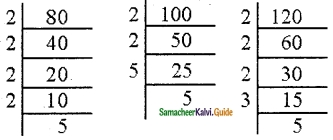80 = 2 × 2 × 2 × 2 × 5
100 = 2 × 2 × 5 × 5
120 = 2 × 2 × 2 × 3 × 5
HCF of 80, 100 and 120 = 2 × 2 × 5 = 20
Volume of the vessel = 20 litres

Question 7.
The traffic lights at three different road junctions change after every 40 seconds, 60 seconds, and 72 seconds respectively. If they changed simultaneously together at 8 a.m at the junctions, at what time will they simultaneously change together again?
Solution:
This is an LCM related problem. So, we need to find the LCM of 40, 60, 72LCM of 40, 60 and 72
= 2 × 2 × 3 × 5 × 2 × 1 × 1 × 3
= 360 seconds
= 6 minutes
The traffic lights will change simultane¬ously again at 8 : 06 am.Question 8.
The LCM of two numbers is 210 and their HCF is 14. How many such pairs are possible.
Solution:
Product of two numbers = LCM × HCF
x × y = 210 × 14
x × y = 2940
(12, 245), (20, 147)
Two pairs are possible.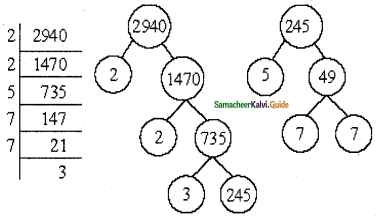Question 9.
The LCM of two numbers is 6 times their HCF. If the HCF is 12 and one of the numbers is 36, then find the other number.
Solution:
36x = 6 × 12 × 12
⇒ $$x=\frac{6 \times 12 \times 12}{36}=24$$Objective Type Questions

Question 10.
Which of the following pairs is co-prime?
(a) 51, 63
(b) 52, 91
(c) 71, 81
(d) 81, 99
Solution:
(c) 71, 81

Question 11.
The greatest four-digit number which is exactly divisible by 8, 9, and 12 is
(a) 9999
(b) 9996
(c) 9696
(d) 9936
Solution:
(d) 9936Question 12.
The HCF of two numbers is 2 and their LCM is 154. If the difference between the numbers is 8, then the sum is
(a) 26
(b) 36
(c) 46
(d) 56
Solution:
(b) 36

Question 13.
Which of the following cannot be the HCF of two numbers whose LCM is 120?
(a) 60
(b) 40
(c) 80
(d) 30
Solution:
(c) 80